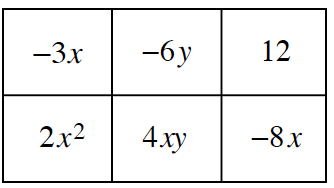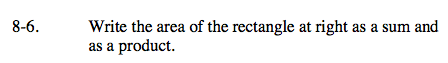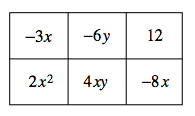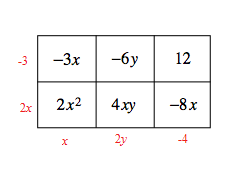### Home > CCA > Chapter 8 > Lesson 8.1.1 > Problem8-6

8-6.Write the area of the rectangle at right as a sum and as a product. Homework Help ✎Find the dimensions of the large rectangle.

To show the area as a product, write the dimensions multiplied together.

To show the area as a sum, add up all of the tile pieces.As a product area = (2x − 3)(x + 2y − 4)
As a sum area = 2x² − 11x + 4xy − 6y + 12